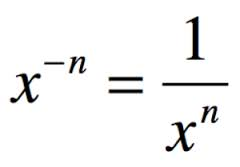# Something I learned this week (oct. 31- nov. 4)

A useful skill we did in class this week was learning how to “FOIL” polynomials> FOIL is an acronym that stands for the First terms of the brackets, Outside terms, Inside terms, and Last terms of the brackets. In the example,$(x^2-5)(y+2)$ the first step would be to multiply the first terms in each bracket,$x^2$ and y to get$x^2y$. Next is to multiply$x^2$ and 2 (the outside terms) with a product of$2x^2$. Then -5 and y for the inside terms to get -5y. And lastly -5 and 2 for the last terms from each bracket to get -10. Our expression now stands as,$x^2y+2x^2-5y-10$ and as there are no like terms, we can’s simplify any further.

# Something I learned this week (Oct. 24-28)

This week, I learned how to use the acronym SOH CAH TOA to help find angles and sides of right angled triangles.
SOH represents “sine, opposite, hypotenuse” So to find the sine, you put the ratio of the opposite side over the hypotenuse. In this example, in respect to angle C, the sine is$\frac{24}{25}$
CAH represents “cosine, adjacent, hypotenuse”  To find the cosine, you put the ratio of the adjacent side over the hypotenuse. In this example, in respect to angle C, the cosine is$\frac{7}{25}$
TOA represents “tangent, opposite, adjacent” To find the tangent, you put the ratio of the opposite side over the adjacent. In this example, in respect to angle C, the tangent is$\frac{7}{24}$# Something I learned this week (oct 10-14)

This week I learned how to convert from imperial to metric, and how to convert within both systems of measurement using unit analysis.# Something I learned this week (oct. 3-7 )

This week I learned how to read a micrometer and a vernier caliper. Both tools of measurement were easy to read after a bit of practice. I found it easier to read the micrometer with the strategy of reading it in dollars and cents.# Something I learned this week

In math this week, I learned how to change a negative exponent into a positive one, so if it was a numerical base, evaluate the question. To do this you flip the base, since it can be written as a fraction of x/1, we flip it to become 1/x and the exponent on x would no longer be negative.# GCF/LCM

To find the GCF, one method is to look at your numbers and find a factor that they both can divide by.

 522 216 864

For the numbers here, I can see that they are both divisible by 9. I write the 9 in the next section below and divide both the numbers and write the quotients beneath the original.

 522 216 864 9 58 24 96

Repeat the process with the next sets of numbers.

 522 216 864 9 58 24 96 2 29 12 48

Once your last set of numbers don’t have a common factor, you multiply all the numbers in the left column together to find the GCF

 522 216 864 9 58 24 96 2 29 12 48

9 x 2= 18

The GCF of 522, 216 and 864 is 18

To find the LCM using this method, you multiply together all of the factors on the left column and the bottom row. (Creating an L shape for the LCM)

 522 216 864 9 58 24 96 2 29 12 48

9 x 2 x 29 x 12 x 48= 300 672

The LCM of 552, 216 and 864 is 300 672

I personally prefer the strategy given in class because it seems more logical to me. I find the numerical process easier to understand when it’s clear cut, rather than drawn out and being shown every single step.